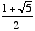index: click on a letter A B C D E F G H I J K L M N O P Q R S T U V W X Y Z A to Z index index: subject areas numbers & symbols sets, logic, proofs geometry algebra trigonometry advanced algebra & pre-calculus calculus advanced topics probability & statistics real world applications multimedia entrieswww.mathwords.com about mathwords website feedback

 Golden Mean Golden Ratio The number, or about 1.61803. The Golden Mean arises in many settings, particularly in connection with the Fibonacci sequence. Note: The reciprocal of the Golden Mean is about 0.61803, so the Golden Mean equals its reciprocal plus one. It is also a root of x2 – x – 1 = 0. Note: The Greek letter phi, φ, is often used as a symbol for the Golden Mean. Occasionally the Greek letter tau, τ, is used as well.   See also